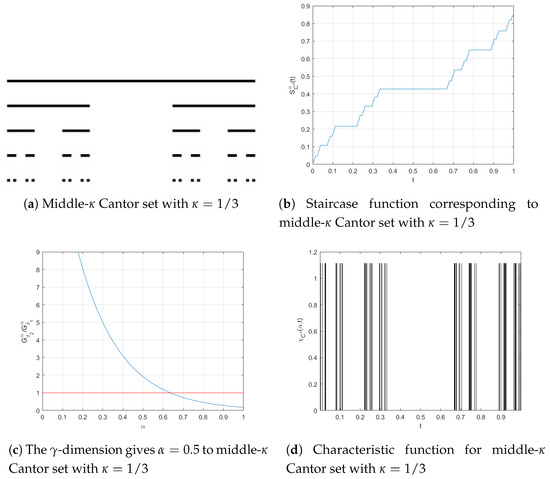Next Article in Journal
Exploration of Filled-In Julia Sets Arising from Centered Polygonal Lacunary Functions
Previous Article in Journal
Cornu Spirals and the Triangular Lacunary Trigonometric System
Open AccessArticle

# Fractal Logistic Equation

byAlireza Khalili Golmankhaneh 1,*andCarlo Cattani 21
Department of Physics, Urmia Branch, Islamic Azad University, Urmia, PO Box 969, Iran
2
Engineering School, DEIM, Tuscia University, Viterbo, Italy and Ton Duc Tang University, HCMC, Ho Chí Minh 758307, Vietnam
*
Author to whom correspondence should be addressed.
Fractal Fract 2019, 3(3), 41; https://doi.org/10.3390/fractalfract3030041
Received: 4 June 2019 / Revised: 2 July 2019 / Accepted: 8 July 2019 / Published: 11 July 2019
In this paper, we give difference equations on fractal sets and their corresponding fractal differential equations. An analogue of the classical Euler method in fractal calculus is defined. This fractal Euler method presets a numerical method for solving fractal differential equations and finding approximate analytical solutions. Fractal differential equations are solved by using the fractal Euler method. Furthermore, fractal logistic equations and functions are given, which are useful in modeling growth of elements in sciences including biology and economics. View Full-Text
Show FiguresFigure 1

MDPI and ACS Style

Khalili Golmankhaneh, A.; Cattani, C. Fractal Logistic Equation. Fractal Fract 2019, 3, 41.